• 一、程序描述 冒泡排序(BubbleSort)简单来讲就是通过对其中的元素按顺序两两进行对比，按照规则不断交换，使得一些元素慢慢被换到后面，就像...冒泡排序一般用于初学计算机程序设计的人理解算法。 算法稳定性：如果相等

一、程序描述
冒泡排序(BubbleSort)简单来讲就是通过对其中的元素按顺序两两进行对比，按照规则不断交换，使得一些元素慢慢被换到后面，就像是水中的气泡慢慢的浮出水面（跑到后面）一样。这是一种简单的基于比较大小的排序算法。冒泡排序利用了不等式传递的思想。

算法原理：如果想把某个数组按照递增顺序排列，那么应该从头开始比较相邻的两个元素，如果后面一个元素比前面一个要大，说明顺序不对，就把他们换个位置，直到所有元素全部交换完成，则排序完成。冒泡排序一般用于初学计算机程序设计的人理解算法。

算法稳定性：如果相等的两个元素相邻，那么根据算法定义不会发生交换，如果相等的两个元素不相邻，那么通过其他的两两交换让他们相邻后也不会发生交换，所以相同元素的顺序不会发生改变，冒泡排序是一种稳定排序算法。

算法优化：
1、冒泡的同时把“水”沉下去。（上浮+下沉）
2、泡泡到顶以后再也不进行扫描。（减少无效扫描）
3、如果检测到一整轮没有发生交换，直接结束排序。

二、程序要点
1、冒泡的同时把“水”沉下去。（上浮+下沉）双向排序

#define _CRT_SECURE_NO_WARNINGS
#include <stdio.h>
void bubble_sort_down(int arr[], int sz)//冒泡排序，双向扫描
{
int i = 0;
int t;
int m;
for (i = 0; i < (sz)/2; i++)//进行元素个数减一次排序,双向排序中可以让排序次数减半
{
int flag = 1;//定义一个整型变量一会用来判断if语句
int j;
int k;
for (j = 0; j < sz - 1 - i; j++)//每次排序次数比较次数减少i个，也就是不再对已经上浮到位的元素进行比较
{
if (arr[j] > arr[j + 1])
{
t = arr[j];
arr[j] = arr[j + 1];
arr[j + 1] = t;
flag = 0;//程序如果没来这，说明这一次扫描没有发生过换位，所以可以直接结束排序
}
}
for (k = sz -1-i; k > 0; k--)//从小到大排
{
if (arr[k] < arr[k - 1])
{
m = arr[k];
arr[k] = arr[k - 1];
arr[k - 1] = m;
flag = 0;
}
}
if (flag == 1)
{
break;
}
}
}
int main()
{
int i = 0;
int arr = { 9,8,7,6,5,4,3,2,1,0 };//排为升序
int sz = sizeof(arr) / sizeof(arr);
bubble_sort_down(arr, sz);//冒泡排序函数
for (i = 0; i < sz; i++)
{
printf("%d\n", arr[i]);
}
return 0;
}

2、泡泡到顶以后再也不对它进行扫描。函数调用

#define _CRT_SECURE_NO_WARNINGS
#include <stdio.h>
void bubble_sort(int arr[],int sz)//冒泡排序，这个代码效率比较低
{
int i = 0;
int t;
for (i = 0; i < sz-1; i++)//进行元素个数减一次排序
{
int j;
for (j = 0; j <sz-1-i; j++)//每次排序次数比较次数减少i个，也就是不再对已经上浮到位的元素进行比较
{
if (arr[j] > arr[j + 1])
{
t = arr[j];
arr[j] = arr[j + 1];
arr[j + 1] = t;
}
else
continue;
}
}
}
int main()
{
int i=0;
int arr = { 9,8,7,6,5,4,3,2,1,0 };//排为升序
int sz = sizeof(arr) / sizeof(arr);
bubble_sort(arr,sz);//冒泡排序函数
for (i = 0; i < sz; i++)
{
printf("%d\n", arr[i]);
}
return 0;
}

3、如果检测到一整轮没有发生交换，直接结束排序。

#define _CRT_SECURE_NO_WARNINGS
#include <stdio.h>
void bubble_sort(int arr[],int sz)//冒泡排序，一定的改进
{
int i = 0;
int t;
for (i = 0; i < sz-1; i++)//进行元素个数减一次排序
{
int flag = 1;//定义一个整型变量一会用来判断if语句
int j;
for (j = 0; j <sz-1-i; j++)//每次排序次数比较次数减少i个，也就是不再对已经上浮到位的元素进行比较
{
if (arr[j] > arr[j + 1])
{
t = arr[j];
arr[j] = arr[j + 1];
arr[j + 1] = t;
flag = 0;//程序如果没来这，说明这一次扫描没有发生过换位，所以可以直接结束排序
}
else
continue;
}
if (flag == 1)
{
break;
}
}
}
int main()
{
int i=0;
int arr = { 9,8,7,6,5,4,3,2,1,0 };//排为升序
int sz = sizeof(arr) / sizeof(arr);
bubble_sort(arr,sz);//冒泡排序函数
for (i = 0; i < sz; i++)
{
printf("%d\n", arr[i]);
}
return 0;
}

展开全文c语言 算法 排序算法 数据结构
• 冒泡排序及其优化（三种优化）

千次阅读 多人点赞 2019-11-17 21:37:28
文章目录普通的冒泡排序优化一：设置标志位优化二：设置结束边界优化三：双向冒泡排序最终优化优化一 + 优化二 + 双向冒泡写在最后参考 普通的冒泡排序 public void bubbleSort(int[] a) { for (int i = 0; i &...

普通的冒泡排序

public void bubbleSort(int[] a) {
for (int i = 0; i < a.length - 1; i++) {    // 外层控制循环次数
for (int j = 0; j < a.length - i - 1; j++) { // 内层保证 a[n-i-1] 是最大的
if (a[j] > a[j+1]) {
swap(a, j, j+1);
}
}
}
}

最简单版本的冒泡排序，没啥好说的，其中 swap 方法用于交换数组元素，之后都会用到：

private void swap(int[] a, int i, int j) {
int temp = a[i];
a[i] = a[j];
a[j] = temp;
}

优化一：设置标志位

可以设置一个标志位，记录上一次排序是否有交换，没有交换就可以提前结束了。

public void bubbleSort_b1(int[] a) {
boolean hasSwap = true;
for (int i = 0; i < a.length - 1; i++) {    // 外层控制循环次数
if (!hasSwap) {   // 上一轮没有交换，提前结束
break;
}
hasSwap = false;
for (int j = 0; j < a.length - i - 1; j++) { // 内层保证 a[n-i-1] 是最大的
if (a[j] > a[j+1]) {
swap(a, j, j+1);
hasSwap = true;
}
}
}
}

优化二：设置结束边界

记录上一次最后交换的位置，作为下一次循环的结束边界。

最后一次比较说明在那之后的元素都已经排好序，无需再比较，可以避免一些无意义的比较。

public void bubbleSort_b2(int[] a) {
boolean hasSwap = true;
int lastSwapIndex = a.length-1;   // 最后一次交换的位置，初始时设为“数组长度 - 1”
for (int i = 0; i < a.length - 1; i++) {    // 外层控制循环次数
if (!hasSwap) {   // 上一轮没有交换，提前结束
break;
}
hasSwap = false;
int end = lastSwapIndex;   // 上一次的最后交换位置作为这次循环的边界
for (int j = 0; j < end; j++) {
if (a[j] > a[j+1]) {
swap(a, j, j+1);
hasSwap = true;
lastSwapIndex = j;  // 更新最后交换位置
}
}
}
}

优化三：双向冒泡排序

双向冒泡排序，又叫鸡尾酒排序（Cocktail Sort）。

它的过程是：先从左往右比较一次，再从右往左比较一次，然后又从左往右比较一次，以此类推。

它是为了优化前面的大部分元素都已经排好序的数组的排序，例如对于数组 [2,3,4,5,6,7,8,1]，如果使用普通的冒泡排序，需要比较七次；而换成双向冒泡排序，只需比较三次。

简单起见，先看下单纯的双向冒泡排序（没有结合前面两种优化）：

public void cocktailSort(int[] a) {
int left = 0;
int right = a.length - 1;

while (left < right) {
for (int i = left; i < right; i++) { // 保证 a[right] 是最大的
if (a[i] > a[i+1]) {
swap(a, i, i+1);
}
}
right--;
for (int i = right; i > left; i--) { // 保证 a[left] 是最小的
if (a[i] < a[i-1]) {
swap(a, i, i-1);
}
}
left++;
}
}

最终优化：优化一 + 优化二 + 双向冒泡

将设置标志位和记录最后一次交换位置的优化思路应用在双向冒泡上，就形成了最终的优化版本：

public void cocktailSort_b(int[] a) {
int left = 0;
int right = a.length - 1;
int lastSwapIndex = 0;      // 记录最后一次交换的位置
boolean hasSwap = false;    // 标志位

while (left < right) {
for (int i = left; i < right; i++) { // 保证 a[right] 是最大的
if (a[i] > a[i+1]) {
swap(a, i, i+1);
hasSwap = true;
lastSwapIndex = i;
}
}
right = lastSwapIndex;  // 将最后一次交换的位置作为右边界
if (!hasSwap) { // 上一轮没有交换，提前结束
break;
}
hasSwap = false;
for (int i = right; i > left; i--) { // 保证 a[left] 是最小的
if (a[i] < a[i-1]) {
swap(a, i, i-1);
hasSwap = true;
lastSwapIndex = i;
}
}
left = lastSwapIndex;  // 将最后一次交换的位置作为左边界
if (!hasSwap) { // 上一轮没有交换，提前结束
break;
}
hasSwap = false;
}
}

写在最后

这篇文章主要是记录了冒泡排序的优化思路和实现方式。没有什么图片说明，可能比较抽象，如果需要看图来理解的话推荐你看下小灰的两篇介绍冒泡排序和鸡尾酒排序的文章。我就是看了小灰的文章才理解了双向冒泡排序优化在哪里。小灰的文章链接我会在下面参考资料中给出。

参考

展开全文冒泡排序
• 快速排序的4种优化

万次阅读 多人点赞 2018-09-15 22:14:28
快速排序优化 优化1：序列长度达到一定大小时，使用插入排序 优化2：尾递归优化 优化3：聚集元素 优化4：多线程处理快排 快排思想 快排算法是基于分治策略的排序算法，其基本思想是，对于输入的数组a[low, ...

快排思想

快排基准的选择

快速排序的优化

优化1：序列长度达到一定大小时，使用插入排序

优化2：尾递归优化

优化3：聚集元素

优化4：多线程处理快排

快排思想

快排算法是基于分治策略的排序算法，其基本思想是，对于输入的数组 a[low, high]，按以下三个步骤进行排序。

(1)分解：以 a[p] 为基准将 a[low: high] 划分为三段 a[low: p-1]，a[p] 和 a[p+1: high]，使得 a[low: p-1] 中任何一个元素小于等于 a[p]， 而 a[p+1: high] 中任何一个元素大于等于 a[p]。

(2)递归求解：通过递归调用快速排序算法分别对 a[low: p-1] 和 a[p+1: high] 进行排序。

(3)合并：由于对 a[low: p-1] 和 a[p+1: high] 的排序是就地进行的，所以在 a[low: p-1] 和 a[p+1: high] 都已排好序后，不需要执行任何计算，a[low: high] 就已经排好序了。

快排基准的选择

快速排序的运行时间与划分是否对称有关。最坏情况下，每次划分过程产生两个区域分别包含n-1个元素和1个元素，其时间复杂度会达到O(n^2)。在最好的情况下，每次划分所取的基准都恰好是中值，即每次划分都产生两个大小为n/2的区域。此时，快排的时间复杂度为O(nlogn)。所以基准的选择对快排而言至关重要。快排中基准的选择方式有以下三种。

(1)固定基准

以下Partition()函数对数组进行划分时，以元素x = a[low]作为划分的基准。

template <class T>
int Partition(T a[], int low, int high)
{
int i = low, j = high+1;
T x = a[low];
while(true)
{
while(a[++i] < x && i < high);
while(a[--j] > x);
if(i >= j)break;
Swap(a[i], a[j]);
}
a[low] = a[j];
a[j] = x;
return j;
}

快排过程一趟：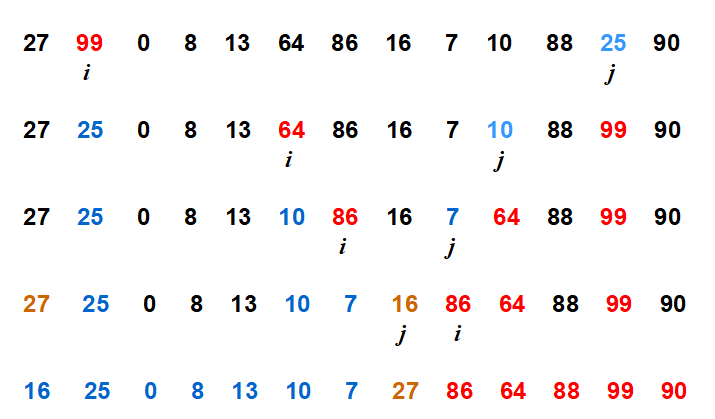快排动图(网上找的动图，其中有一个基准为 6 的标识错误。虽然基准选择方法不一样，但排序过程还是一样的)：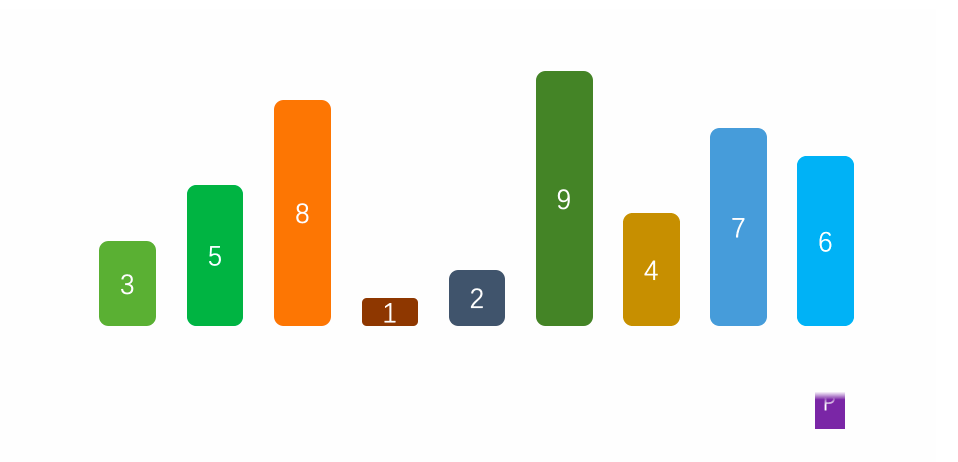如果数组元素是随机的，划分过程不产生极端情况，那么程序的运行时间不会有太大的波动。如果数组元素已经基本有序时，此时的划分就容易产生最坏的情况，即快速排序变成冒泡排序，时间复杂度为O(n^2)。

例如：序列以固定基准进行快排时。

第一趟：

第二趟：

第三趟：

第四趟：

程序中要用的函数：(1)C++可以使用以下方法产生随机数，而单纯的使用rand()%M产生的是伪随机数。

#define M 100
srand(time(0));
for(int i = 0; i < M; i++)
a[i] = rand()%(M);

(2)方法一：获得程序片段运行的时间：

clock_t start_time = clock();
//程序代码片段
clock_t end_time = clock();
cout<<"Runing time is:"<<static_cast<double>(end_time-start_time)/CLOCKS_PER_SEC*1000<<"ms"<<endl;

方法二：获得程序片段运行的时间：

#include<sys/time.h>
int gettimeofday(struct timeval*tv,struct timezone *tz )
struct timeval
{
long tv_sec;/*秒*/
long tv_usec;/*微妙*/
};
struct timezone
{
int tz_minuteswest;
/*和greenwich时间差*/
int tz_dsttime;
};

例如：

int main()
{
float Time=0;
struct timeval start;
struct timeval end;

gettimeofday(&start,NULL); //gettimeofday(&start,&tz);结果一样
//程序片段
gettimeofday(&end,NULL);

Time=(end.tv_sec-start.tv_sec)*1000000+(end.tv_usec-start.tv_usec);//微秒
cout << Time <<endl;
return 0;
}

完整代码如下：

#include <iostream>
#include <time.h>
#include <stdlib.h>
#include <cstdlib>
#define M 1000000
using namespace std;

template <class T>
void Print(T a[], int n, int m)
{
for(int i = n; i < m; i++)
{
cout << "[" << a[i] << "]";
}
cout <<endl;
}

template <class T>
void Swap(T &a, T &b)
{
T asd;
asd = a;
a = b;
b = asd;
}

template <class T>
int Partition(T a[], int p, int r)
{
int i = p, j = r+1;
T x = a[p];
while(true)
{
while(a[++i] < x && i < r);
while(a[--j] > x);
if(i >= j)break;
Swap(a[i], a[j]);
}
a[p] = a[j];
a[j] = x;
return j;
}

template <class T>
void QuickSort(T a[], int p, int r)
{
if(p < r)
{
int q = Partition(a, p, r);
QuickSort(a, p, q-1);
QuickSort(a, q+1, r);
}
}

int a[M] = {0};
int main()
{
srand(time(0));
for(int i = 0; i < M; i++)
//a[i] = i+1;            //设置升序数组
a[i] = rand()%(M);       //设置随机数组
//if(i < M/2-1)          //设置重复数组
//	a[i] = 5;
//else
//	a[i] = 10;
//在检验数据的时候可以用Print()函数将其打印出来
//Print(a, 0, M);
clock_t start_time = clock();
QuickSort(a, 0, M-1);
clock_t end_time = clock();
cout<<"Runing time is:"<<static_cast<double>(end_time - start_time)/CLOCKS_PER_SEC*1000<<"ms"<<endl;
//Print(a, 0, M);
return 0;
}

p.s：(1)在Codeblocks下处理升序数组时，元素最好设置少一点。设置的太大可能会出现下图提示：(2)重复数组中的元素值只有两个。

(3)随机数组(较多重复元素)的设置是：a[i] = rand()%(M/100);。

数据如下：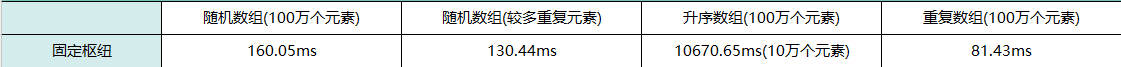固定基准对升序数组的分割极其糟糕，排序时间特别长，所以只设置了10万个元素。

(2)随机基准

在待排数组有序或基本有序的情况下，选择使用固定基准影响快排的效率。为了解决数组基本有序的问题，可以采用随机基准的方式来化解这一问题。算法如下：

int Random(int a[], int low, int high)//在low和high间随机选择一元素作为划分的基准
{
srand(time(0));
int pivot = rand()%(high - low) + low;
Swap(a[pivot],a[low]); //把随机基准位置的元素和low位置元素互换
return a[low];
}

此时，原来Partition()函数里的T x = a[low];相应的改为T x = Random(a, low, high);

虽然使用随机基准能解决待排数组基本有序的情况，但是由于这种随机性的存在，对其他情况的数组也会有影响(若数组元素是随机的，使用固定基准常常优于随机基准)。随机数算法(Sherwood算法)能有效的减少升序数组排序所用的时间，数组元素越多，随机数算法的效果越好。可以试想，上述升序数组中有10万个元素而且各不相同，那么在第一次划分时，基准选的最差的概率就是十万分之一。当然，选择最优基准的概率也是十万分之一，随机数算法随机选择一个元素作为划分基准，算法的平均性能较好，从而避免了最坏情况的多次发生。许多算法书中都有介绍随机数算法，因为算法对程序的优化程度和下面所讲的三数取中方法很接近，所以我只记录了一种方法的运行时间。

(3)三数取中

由于随机基准选取的随机性，使得它并不能很好的适用于所有情况(即使是同一个数组，多次运行的时间也大有不同)。目前，比较好的方法是使用三数取中选取基准。它的思想是：选取数组开头，中间和结尾的元素，通过比较，选择中间的值作为快排的基准。其实可以将这个数字扩展到更大(例如5数取中，7数取中等)。这种方式能很好的解决待排数组基本有序的情况，而且选取的基准没有随机性。

例如：序列，三个元素分别是、、，此时选择作为基准。

第一趟：

三数取中算法如下：

int NumberOfThree(int arr[],int low,int high)
{
int mid = low + ((high - low) >> 1);//右移相当于除以2

if (arr[mid] > arr[high])
{
Swap(arr[mid],arr[high]);
}
if (arr[low] > arr[high])
{
Swap(arr[low],arr[high]);
}
if (arr[mid] > arr[low])
{
Swap(arr[mid],arr[low]);
}
//此时，arr[mid] <= arr[low] <= arr[high]
return arr[low];
}

同理，Partition()函数里的T x = a[low];相应的改为T x = NumberOfThree(a, low, high);

数据如下：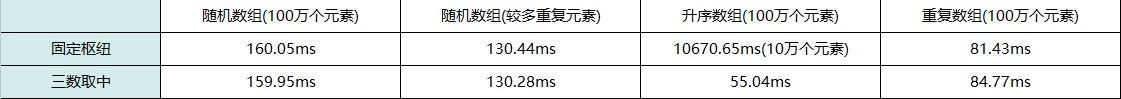三数取中(随机数算法效果相同)在处理升序数组时有质的飞越，而且处理的还是100万个元素。

快速排序的优化

优化1：序列长度达到一定大小时，使用插入排序

当快排达到一定深度后，划分的区间很小时，再使用快排的效率不高。当待排序列的长度达到一定数值后，可以使用插入排序。由《数据结构与算法分析》(Mark Allen Weiness所著)可知，当待排序列长度为5~20之间，此时使用插入排序能避免一些有害的退化情形。

template <class T>
void QSort(T arr[],int low,int high)
{
int pivotPos;
if (high - low + 1 < 10)
{
InsertSort(arr,low,high);
return;
}
if(low < high)
{
pivotPos = Partition(arr,low,high);
QSort(arr,low,pivotPos-1);
QSort(arr,pivotPos+1,high);
}
}

完整代码如下：

/*
次快排代码采用了  三数取中&插入排序
*/
#include <iostream>
#include <time.h>
#include <stdlib.h>
using namespace std;

#define M 1000000
int NumberOfThree(int arr[],int low,int high);

template <class T>
void Print(T a[], int n)
{
for(int i = 0; i < n; i++)
{
cout << "[" << a[i] << "]";
}
cout <<endl;
}

template <class T>
void Swap(T &a, T &b)
{
T asd;
asd = a;
a = b;
b = asd;
}

template <class T>
int Partition(T a[], int p, int r)
{
int i = p, j = r+1;
T x = NumberOfThree(a, p, r);
while(true)
{
while(a[++i] < x && i < r);
while(a[--j] > x);
if(i >= j)break;
Swap(a[i], a[j]);
}
a[p] = a[j];
a[j] = x;
return j;
}

void InsertSort(int arr[], int m, int n)
{
int i, j;
int temp; // 用来存放临时的变量
for(i = m+1; i <= n; i++)
{
temp = arr[i];
for(j = i-1; (j >= m)&&(arr[j] > temp); j--)
{
arr[j + 1] = arr[j];
}
arr[j + 1] = temp;
}
}

int NumberOfThree(int arr[],int low,int high)
{
int mid = low + ((high - low) >> 1);

if (arr[mid] > arr[high])
{
Swap(arr[mid],arr[high]);
}
if (arr[low] > arr[high])
{
Swap(arr[low],arr[high]);
}
if (arr[mid] > arr[low])
{
Swap(arr[mid],arr[low]);
}
//此时，arr[mid] <= arr[low] <= arr[high]
return arr[low];
}

template <class T>
void QSort(T arr[],int low,int high)
{
int pivotPos;
if (high - low + 1 < 10)
{
InsertSort(arr,low,high);
return;
}
if(low < high)
{
pivotPos = Partition(arr,low,high);
QSort(arr,low,pivotPos-1);
QSort(arr,pivotPos+1,high);
}
}

int a[M] = {0};
int main()
{
srand(time(0));
for(int i=0;i<M;i++)
//a[i] = i+1;              //设置升序数组
a[i] = rand()%(M);         //设置随机数组
//if(i < M/2-1)            //设置重复数组
//	a[i] = 1;
//else
//	a[i] = 10;
//Print(a, M);
clock_t start_time = clock();
QSort(a, 0, M-1);
clock_t end_time = clock();
cout<<"Runing time is:"<<static_cast<double>(end_time - start_time)/CLOCKS_PER_SEC*1000<<"ms"<<endl;
//Print(a, M);
return 0;
}

数据如下：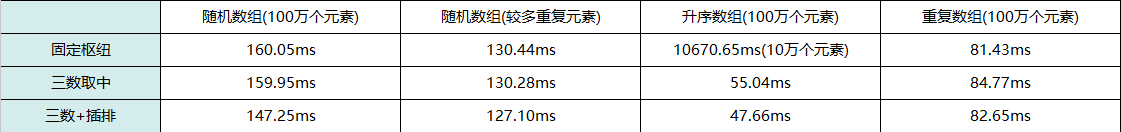如上所述，在划分到很小的区间时，里面的元素已经基本有序了，再使用快排，效率就不高了。所以，在结合插入排序后，程序的执行效率有所提高。

优化2：尾递归优化

快排算法和大多数分治排序算法一样，都有两次递归调用。但是快排与归并排序不同，归并的递归则在函数一开始， 快排的递归在函数尾部，这就使得快排代码可以实施尾递归优化。使用尾递归优化后，可以缩减堆栈的深度，由原来的O(n)缩减为O(logn)。

尾递归概念：

如果一个函数中所有递归形式的调用都出现在函数的末尾，当递归调用是整个函数体中最后执行的语句且它的返回值不属于表达式的一部分时，这个递归调用就是尾递归。尾递归函数的特点是在回归过程中不用做任何操作，这个特性很重要，因为大多数现代的编译器会利用这种特点自动生成优化的代码。

尾递归原理：

编译器检测到一个函数调用是尾递归的时候，它就覆盖当前的活动记录而不是在栈中去创建一个新的。编译器可以做到这点，因为递归调用是当前活跃期内最后一条待执行的语句，于是当这个调用返回时栈帧中并没有其他事情可做，因此也就没有保存栈帧的必要了。通过覆盖当前的栈帧而不是在其之上重新添加一个，这样所使用的栈空间就大大缩减了，这使得实际的运行效率会变得更高。

代码如下：

#include <bits/stdc++.h>
using namespace std;

int fact(int n)             //线性递归
{
if (n < 0)
return 0;
else if(n == 0 || n == 1)
return 1;
else
return n * fact(n - 1);
}

int facttail(int n, int a)   //尾递归
{
if (n < 0)
return 0;
else if (n == 0)
return 1;
else if (n == 1)
return a;
else
return facttail(n - 1, n * a);
}

int main()
{
int a = fact(5);
int b = facttail(5, 1);
cout << "A:" << a <<endl;
cout << "B:" << b <<endl;
}

示例中的函数是尾递归的，因为对facttail的单次递归调用是函数返回前最后执行的一条语句。在facttail中碰巧最后一条语句也是对facttail的调用，但这并不是必需的。换句话说，在递归调用之后还可以有其他的语句执行，只是它们只能在递归调用没有执行时才可以执行尾递归是极其重要的，不用尾递归，函数的堆栈耗用难以估量，需要保存很多中间函数的堆栈。比如f(n, sum) = f(n-1) + value(n) + sum; 会保存n个函数调用堆栈，而使用尾递归f(n, sum) = f(n-1, sum+value(n)); 这样则只保留后一个函数堆栈即可，之前的可优化删去。

代码当n=5时，线性递归的递归过程如下:

fact(5)
{5*fact(4)}
{5*{4*fact(3)}}
{5*{4*{3*fact(2)}}}
{5*{4*{3*{2*fact(1)}}}}
{5*{4*{3*{2*1}}}}
{5*{4*{3*2}}}
{5*{4*6}}
{5*24}
120

而尾递归的递归过程如下:

facttail(5,1)
facttail(4,5)
facttail(3,20)
facttail(2,60)
facttail(1,120)
120

关于尾递归及快排尾递归优化可以看这篇博文：尾递归及快排尾递归优化 ，其中包含了上述阶乘问题、快排尾递归优化和Gdb调试等内容。

在Codeblocks里运行快排代码处理升序数组，一个进行尾递归优化，而另一个不变。没有使用尾递归的代码处理4万个数组元素时，由于超过了栈的深度，程序会异常结束。而使用了尾递归的代码，就算处理10万个数组元素，也不会出现异常(结合三数取中，可以处理100万个数组元素)。

2018年10月2日补充：结合我的另一篇博文《内存四区》，对上述问题有更全面的认识。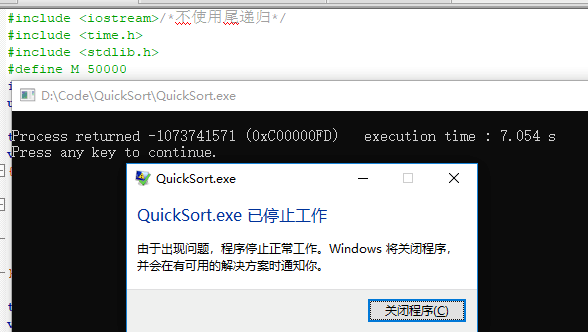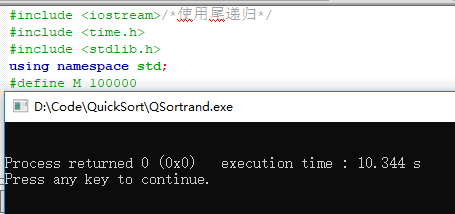快排尾递归代码如下：

template <class T>
void QSort(T arr[],int low,int high)
{
int pivotPos;
if (high - low + 1 < 10)
{
InsertSort(arr,low,high);
return;
}
while(low < high)
{
pivotPos = Partition(arr,low,high);
QSort(arr,low,pivotPos-1);
low = pivotPos + 1;
}
}

第一次递归以后，变量low就没有用处了， 也就是说第二次递归可以用迭代控制结构代替。快排尾递归过程如下，纵向是递归，横向是迭代。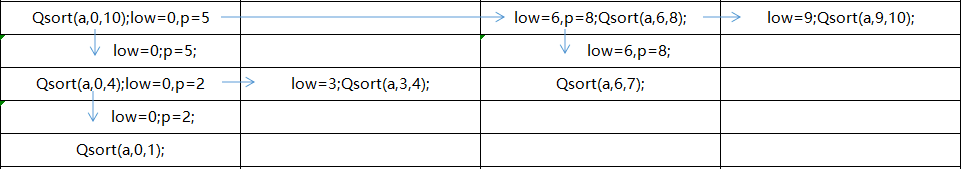数据如下：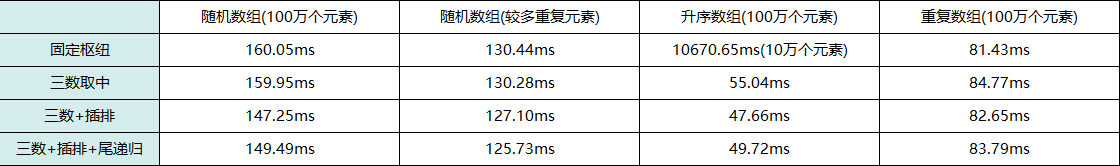对递归的优化，主要是为了减少栈深度。在处理随机数组时，(三数取中+插排+尾递归)的组合并不一定比(三数取中+插排)的效率高。

优化3：聚集元素

聚集元素的思想：在一次分割结束后，将与本次基准相等的元素聚集在一起，再分割时，不再对聚集过的元素进行分割。具体过程有两步，①在划分过程中将与基准值相等的元素放入数组两端，②划分结束后，再将两端的元素移到基准值周围。

普通过程例如： 由三数取中可得基准为

第一趟：         

第二趟：         

第三趟：         

第四趟：         

聚集相同元素：

第一步：         

第二步：         

接下来是对     和 进行快排。(具体过程可以按照以下代码走一遍)

template <class T>
void QSort(T arr[],int low,int high)
{
int first = low;
int last = high;

int left = low;
int right = high;

int leftLen = 0;
int rightLen = 0;

if (high - low + 1 < 10)
{
InsertSort(arr,low,high);
return;
}

//一次分割
int key =  NumberOfThree(arr,low,high);//使用三数取中选择枢轴

while(low < high)
{
while(high > low && arr[high] >= key)
{
if (arr[high] == key)//处理相等元素
{
Swap(arr[right],arr[high]);
right--;
rightLen++;
}
high--;
}
arr[low] = arr[high];
while(high > low && arr[low] <= key)
{
if (arr[low] == key)
{
Swap(arr[left],arr[low]);
left++;
leftLen++;
}
low++;
}
arr[high] = arr[low];
}
arr[low] = key;

//一次快排结束，把与基准相等的元素移到基准周围
int i = low - 1;
int j = first;
while(j < left && arr[i] != key)
{
Swap(arr[i],arr[j]);
i--;
j++;
}
i = low + 1;
j = last;
while(j > right && arr[i] != key)
{
Swap(arr[i],arr[j]);
i++;
j--;
}
QSort(arr,first,low - 1 - leftLen);
QSort(arr,low + 1 + rightLen,last);
}

聚集元素第一步：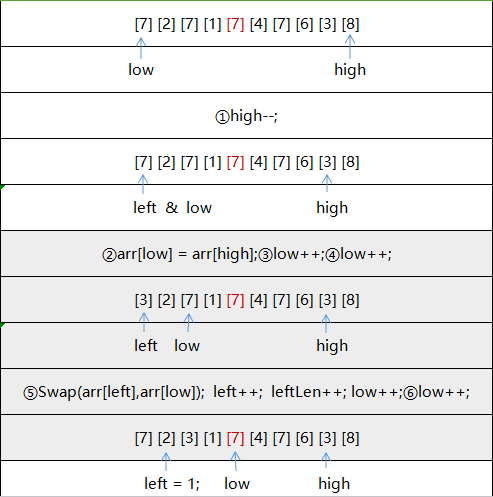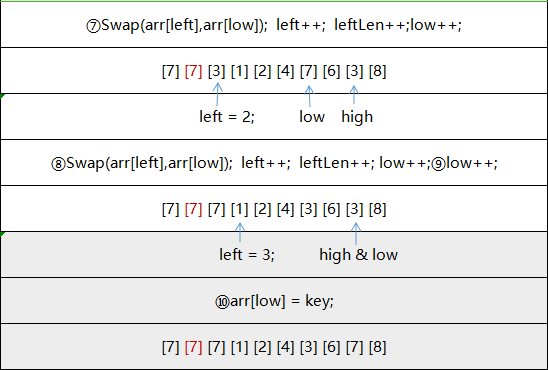聚集元素第二步：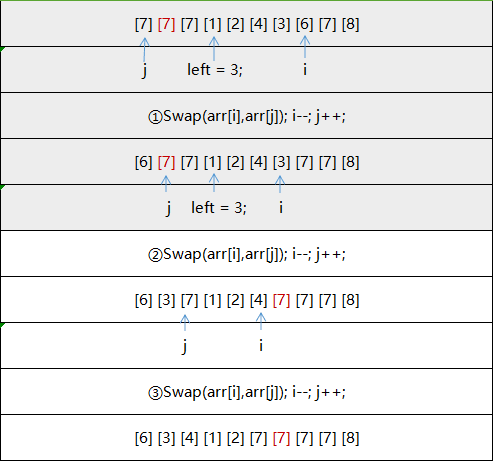下一次就是对     进行快排。当划分区间达到插入排序的要求时，就使用插入排序完成后续工作，所以进入插入排序那一段代码是停止继续递归的标志。

数据如下：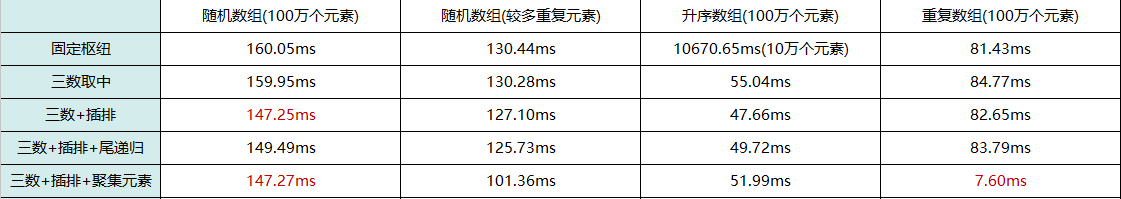从上表中可以看到，通过对快排聚集元素的优化，在处理数组中的重复元素时有很大的提升。而对于升序数组而言，因为其本身就是有序的，而且没有重复元素，所以结果没有(三数取中+插排)效率高。

优化4：多线程处理快排

分治法的基本思想是将一个规模为n的问题分解为k个规模较小的子问题，这些子问题互相独立且与原问题相同。求解这些子问题，然后将各子问题的解合并，从而得到的原问题的解。由此，在处理快排的时候，可以使用多线程提高排序的效率。

要使用的函数：

第一个参数是一个整数类型，它表示的是资源描述符，实际上，Linux上几乎所有的资源标识符都是一个整型数。第二个attr参数用于设置新线程的属性。给它传递NULL表示使用默认线程属性。start_routine和arg参数分别指定新线程将运行的函数及其参数。

函数执行成功返回 0，执行失败则返回一个错误号，我们可以通过该错误号获取相关的错误信息。
第二个参数：用于指定屏障的细节参数，我们这里可以暂且不去管它，如果我们传入NULL，那么系统将按照默认情况处理。
第三个参数：设计屏障等待的最大线程数目。

当一个线程需要等待其它多个线程结束时，调用该函数。
原型：

函数执行成功返回 0，执行失败则返回一个错误码，我们可以通过该错误码获取相关的错误信息。

代码如下：

#include <cstdio> /*三数取中+插入+聚集元素+多线程组合  &&  三数取中+插入+尾递归+多线程组合*/
#include <iostream>
#include <stdlib.h>
#include <sys/time.h>
using namespace std;

const long MAX = 1000000L;                          //数组中最大数
const long long MaxNumber = 1000000L;               //排序数
const int thread = 4;                               //线程数
const long NumberOfSort = MaxNumber / thread;       //每个线程排序的个数

int array_a[MaxNumber];
int array_b[MaxNumber];                             //合并后，由b数组记录最终序列

void initial()   //数组初始化函数
{
srand(time(0));
for(int i = 0; i < MaxNumber; ++i)
array_a[i] = rand()%(MAX);
//if(i < MaxNumber/2)
//    array_a[i] = 5;
//else
//   array_a[i] = 10;
//array_a[i] = i+1;
}

template <class T>
void Print(T a[], int n)
{
for(int i = 0; i < n; i++)
{
cout << "[" << a[i] << "]";
}
cout <<endl;
}

template <class T>
void Swap(T &a, T &b)
{
T asd;
asd = a;
a = b;
b = asd;
}

void InsertSort(int arr[],int start,int end)
{
int low,high,median,tmp;
for(int i = start+1;i<= end;i++)
{
low = start;
high = i-1;

while(low <= high)
{
median = (low + high) /2;
if(arr[i] < arr[median])
{
high = median -1;
}else
{
low = median + 1;
}
}

tmp = arr[i];

for(int j = i-1;j>high;j--)
{
arr[j+1] = arr[j];
}
arr[high+1] = tmp;
}
}

int NumberOfThree(int arr[],int low,int high)
{
int mid = low + ((high - low) >> 1);
if (arr[mid] > arr[high])
{
Swap(arr[mid],arr[high]);
}
if (arr[low] > arr[high])
{
Swap(arr[low],arr[high]);
}
if (arr[mid] > arr[low])
{
Swap(arr[mid],arr[low]);
}
return arr[low];
}

template <class T>
int Partition(T a[], int p, int r)
{
int i = p, j = r+1;
T x = NumberOfThree(a, p, r);
while(true)
{
while(a[++i] < x && i < r);
while(a[--j] > x);
if(i >= j)break;
Swap(a[i], a[j]);
}
a[p] = a[j];
a[j] = x;
return j;
}

#if 1   //有聚集元素的快排
template <class T>
void QSort(T arr[],int low,int high)
{
int first = low;
int last = high;
int left = low;
int right = high;
int leftLen = 0;
int rightLen = 0;

if (high - low + 1 < 6)
{
InsertSort(arr,low,high);
return;
}

//一次分割
int key = NumberOfThree(arr,low,high);//使用三数取中法选择枢轴

while(low < high)
{
while(high > low && arr[high] >= key)
{
if (arr[high] == key)//处理相等元素
{
Swap(arr[right],arr[high]);
right--;
rightLen++;
}
high--;
}
arr[low] = arr[high];
while(high > low && arr[low] <= key)
{
if (arr[low] == key)
{
Swap(arr[left],arr[low]);
left++;
leftLen++;
}
low++;
}
arr[high] = arr[low];
}
arr[low] = key;

//一次快排结束
//把与枢轴key相同的元素移到枢轴最终位置周围
int i = low - 1;
int j = first;
while(j < left && arr[i] != key)
{
Swap(arr[i],arr[j]);
i--;
j++;
}
i = low + 1;
j = last;
while(j > right && arr[i] != key)
{
Swap(arr[i],arr[j]);
i++;
j--;
}
QSort(arr,first,low - 1 - leftLen);
QSort(arr,low + 1 + rightLen,last);
}
#endif

#if 0     //没有聚集元素的快排
template <class T>
void QSort(T arr[],int low,int high)
{
int pivotPos;
if (high - low + 1 < 10)
{
InsertSort(arr,low,high);
return;
}

while(low < high)
{
pivotPos = Partition(arr,low,high);
QSort(arr,low,pivotPos-1);
low = pivotPos + 1;
}
}
#endif // 1

void* work(void *arg)  //线程排序函数
{
long length = (long)arg;
QSort(array_a, length, length + NumberOfSort - 1);
}

void meger()        //最终合并函数
{
for (int i = 0; i < thread; ++i)
{
index[i] = i * NumberOfSort;
}

for(long i = 0; i < MaxNumber; ++i)
{
long min_index;
long min_num = MAX;
for(int j = 0; j < thread; ++j)
{
if((index[j] < (j + 1) * NumberOfSort)&& (array_a[index[j]] < min_num))
{
min_index = j;
min_num = array_a[index[j]];
}
}
array_b[i] = array_a[index[min_index]];
index[min_index]++;
}
}

int main(int argc, char const *argv[])
{
initial();
//Print(array_a,MaxNumber);
struct timeval start, end;
gettimeofday(&start, NULL);

for(int i = 0; i < thread; ++i)
pthread_create(&ptid, NULL, work, (void *)(i * NumberOfSort));

meger();

gettimeofday(&end, NULL);
long long s_usec = start.tv_sec * 1000000 + start.tv_usec;
long long e_usec = end.tv_sec * 1000000 + end.tv_usec;

double Time = (double)(e_usec - s_usec) / 1000.0;
printf("sort use %.4f ms\n", Time);
//Print(array_b, MaxNumber);
return 0;
}

上传完这段代码，同学告诉我说，这段代码在Linux和Codeblocks里运行的时间不一样(本篇博文的数据都是在Codeblocks上测得的)。然后我立马就测试了一下，发现这之间存在误差，初步猜测是由于编译器引起的。由于我不是双系统，是在虚拟机上运行的Linux系统，这可能是造成误差原因之一(个人认为可以忽略误差，虽然每组数据在不同环境下平均运行时间有差距，但其整体优化的方向是不变的)。

数据如下：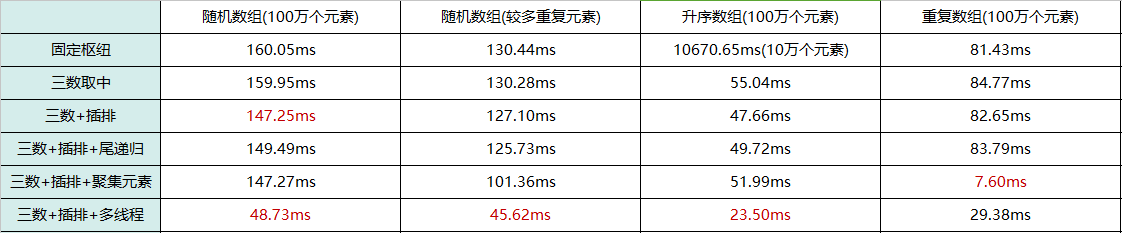从上表可以看出，结合了多线程的快排(三数+插排+多线程)在处理前三种数组时都有明显的提升。重复数组处理时间增加的原因是：聚集元素在处理重复数组时的表现已经很好了，因为在多线程的组合中，各个线程排完序后要合并，所以增加了(三数+插排+多线程)这一组合的排序时间。因为时间原因，以上的数据，是运行相应代码10次所取得平均值。如果想要得到更精确的数据，需要大量的运行上述代码(即使存在一些不稳定的数据，也不会影响到代码优化的方向)。PS.以上程序运行时间还与个人所使用的电脑配置有关。

参考：

http://blog.csdn.net/zuiaituantuan/article/details/5978009

https://blog.csdn.net/hacker00011000/article/details/52176100

https://baike.baidu.com/item/%E5%B0%BE%E9%80%92%E5%BD%92/554682

https://blog.csdn.net/qq_25425023/article/details/72705285

展开全文数据结构 快速排序 尾递归 多线程
• 一、排序算法   a. 一次扫描性能高，减少了io随机请求的次数   b. 排序操作是在内存(sort_buffer)里面进行的， 先select结果再进行排序，如果结果值大于max_length...二、优化思路   a. 尽量减少io的读取 max_
一、排序算法

a.  一次扫描性能高，减少了io随机请求的次数
b.  排序操作是在内存(sort_buffer)里面进行的， 先select结果再进行排序，如果结果值大于max_length_for_sort_data 阀值设置需要二次io读取
c.  关联查询会产生临时表再进行order by

二、优化思路
a.  尽量减少io的读取 max_length_for_sort_data  阀值设置
b.  尽量使用有序排序输出。 运用覆盖索引、主键索引
c.  控制select 字段数，不需要的字段没必要输入
d.   …….

一次扫描算法（4.1版本以后支持）
1)、根据条件取出所有字段信息
2)、在sort_buffer中进行排序, 如果sort_buffer较小，产生临时表，在临时表存储排序结果
选用一次排序扫描还是二次排序扫描， 需要根据参数来： max_length_for_sort_data
如果取的值 大于 max_length_for_sort_data 设置的值，采用二次扫描。
两个参数：
max_length_for_sort_data:  确定选用哪种扫描策略
sort_buffer:   排序过程中确定是否需要创建临时表存储排序结果。每个线程共享。

两次扫描算法
1)、根据条件取出排序字段和行指针信息
2)、在sort_buffer中进行排序, 如果sort_buffer较小，产生临时表，在临时表存储排序结果
3)、根据排序结果的行号去主索引结构中获取数据
例如：select * from t where filed=‘abc’ order by field2
此语句会经过2次扫描
第1次搜索出符合条件的记录
第2次根据行号去获取字段
如果field字段上有索引，解析规则：use index, Using filesort
如果field字段上没有索引，则解析规则：Using where; Using filesort
说明：order by与索引无关; （除覆盖索引除外）
展开全文排序算法 mysql
• 冒泡排序是非常好理解的，以从小到大排序为例，每一轮排序就找出未排序序列中最大值放在最后。冒泡排序
• 搭建搜索功能不难，难的是如何提高搜索质量，帮助用户快速找到心中所想的内容或商品，那么搜索结果的相关性排序则是影响用户体验最关键的一环，本文通过阿里云开放搜索电商行业解决方案和大家聊一聊如何优化排序结果...搜索引擎 搜索引擎优化 云搜索
• 导读：搭建搜索功能不难，难的是如何提高搜索质量，帮助用户快速找到心中所想的内容或商品，那么搜索结果的相关性排序则是影响用户体验最关键的一环，本文通过电商行业的实际业务需求结合阿里云的开放搜索系统的解决...搜索引擎 算法 大数据 人工智能
• 从零开始理解冒泡排序

千次阅读 2018-08-11 23:28:02
冒泡排序是一种基础的入门级别的算法，它的效率比较低，但是理解它的好处是让我们对算法有初步的了解，理解了冒泡排序，不仅仅是让我们写出一个简单算法，完成入门，笔者认为写出一段程序除了能够给我们带来收入以外...Java 算法 编程入门
• 前言：关于 快速排序算法的相关理解，本文借鉴了 啊哈磊 老师的《常用排序——快速排序》 ，在此向作者 致敬，写的挺好。 目录 一、冒泡排序 二、快速排序 三、小结 一、冒泡排序 冒泡排序是各种教材中 ...
• 冒泡排序的双重循环理解

千次阅读 多人点赞 2019-05-13 00:52:24算法学习 冒泡排序理解 奇思妙想
• 十大经典排序算法详解及优化

千次阅读 多人点赞 2018-06-10 17:13:52
包括：交换排序（冒泡、快速排序）插入排序（简单插入排序、希尔排序）选择排序（简单选择排序、堆排序）归并排序（二路，多路） 线性时间非比较类排序： 不通过比较来决定元素间的相对次序，可以突破基于比...排序 数据结构
• /** * * @author Fly */ public class BubbleSort { ... //不加思考写的交换排序， //但不是冒泡排序，比较的不是相邻的元素 public int[] bubbleSort(int[] a) { int size = a.length; for (int i = 0; i ; i
• 冒泡排序优化后

千次阅读 2021-03-21 19:45:45
import java.util.Arrays; /** * @创建人 wdl * @创建时间 2021/3/21 * @描述 ...public class BubbleSort { public static void main... //为了容易理解，我们吧冒泡排序的演变过程给大家展示 //第一趟排序，就排序算法 冒泡排序 优化
• 插入排序算法是所有排序方法中最简单的一种算法，其主要的实现思想是将数据按照一定的顺序一个一个的插入到有序的表中，最终得到的序列就是已经排序好的数据。 直接插入排序是插入排序算法中的一种，采用的方法是：...插入排序 数据结构 算法
• 快速排序， 是最经典的排序算法之一。快速排序拥有良好的时间复杂度，平均为O(nlog2n)O(nlog_{2}n)，最差为O(n2)O(n^2)。在这里，我们不妨略略深入讨论一下快速排序：时间复杂度分析首先说平均时间复杂度。以比较...快速排序 三路划分 快排优化
• 快速排序复杂度证明 及优化

千次阅读 2018-04-02 22:19:08
快排是分治的思想，分解成小问题，解决(排序)，合并(排序过程都是在一个数组上直接操作，不用合并的过程)。快速排序复杂度最坏情况(O(n^2))证明：最坏情况下就是对已经排好序的序列操作，假设是从小到大，那么last就...排序算法
• C 冒泡排序 冒泡排序的优化

千次阅读 2016-01-07 14:33:24
本文包含冒泡排序的三种实现方式 分别为冒泡排序初级版,升级版,终级版(自己起的名字) 使用时只要使用终极版就本以了,终级版为升级版的优化版本 至于初极版和升级版只是为了帮助理解 冒泡排序的时间复杂度为O(n²)C 冒泡排序 冒泡排序优化
• 冒泡排序算法详解及优化

万次阅读 2017-09-29 10:49:12
排序原理：比较两个相邻的元素，将值大的元素交换至右端，小的元素向上冒泡，这也是冒泡算法名称的由来。 排序流程如下： 1）对数组中的各数据，依次比较相邻的两个元素大小，即第0和第1个比较，第1个和第2个...冒泡排序 java 算法
• 二叉排序树的定义和性质 二叉排序树又称二叉排序树。它或者是一个空树，或者是一个具有下列性质的二叉树： 若它的左子树不空，则左子树上所有节点的值均小于它的根结构的值 若它的右子树不空，则右子树上所有结点的...
• 计数排序的理解与实现

万次阅读 2015-07-16 16:03:43
计数排序是一种非基于比较的排序算法，其空间复杂度和时间复杂度均为O(n+k)计数排序
• 搭建搜索功能不难，难的是如何提高搜索质量，帮助用户快速找到心中所想的内容或商品，那么搜索结果的相关性排序则是影响用户体验最关键的一环，本文通过阿里云开放搜索电商行业解决方案和大家聊一聊如何优化排序结果...大数据 算法 人工智能
• 1.概述 快速排序是冒泡排序的改进算法。...通过一趟排序将待排序数据分割成独立的两个子序列，其中左边的子序列均比右边的子序列中的元素小，然后分别对左右两个子序列继续进行排序，达到整个序列有序的...
• hive 全排序优化

千次阅读 2012-03-19 15:56:39
排序 Hive的排序关键字是SORT BY，它有意区别于传统数据库的ORDER BY也是为了强调两者的区别–SORT BY只能在单机范围内排序。考虑以下表定义： CREATE TABLE if not exists t_order( id int, -- 订单...优化 table hadoop string list
• 冒泡排序算法-C语言实现及针对性优化详解

千次阅读 多人点赞 2021-03-22 20:15:42
提示：本文是为C语言排序算法小萌新科普，其他语言的朋友可以参考，大佬“非礼勿视”对于冒泡排序，我们应该对它的思想进行理解，作为排序算法学习的引导，让我们的思维更加开阔。 文章目录前言一、冒泡排序算法是...c语言 排序算法 程序设计 编程语言 数组
• 白话解析冒泡排序优化

千次阅读 2019-11-01 14:32:10
冒泡排序就是每2个相邻的数据进行比较后，按照升降序进行交换，每次排序至少会让一个最大或最小的元素（默认从左至右排序，升序为最大元素... 我们首先看下优化前的冒泡排序: public static int[] bubbleSort(in...冒泡排序 优化 深度思考
• 在我们写一些简单的程序中，对一组数据进行简单的有序的排列是经常会遇到的，所以我们必须熟悉几种基本的算法。...形象的可以理解为：水中上升的气泡，在上升的过程中，气泡越来越小，不断比较相邻的元素，将小的往后调冒泡的时间复杂度
• 冒泡排序的过程就如同它的名字一样，每次冒泡的过程会将元素中最大/小的一个数冒出来，这样最后的一个元素就会是最大/小的元素，下一次冒泡过程就可以对前n-1个再进行排序，n趟过程下来整个序列就变成有序的了。...java 八大排序算法
• 【算法理解】—— 快速排序（三向切分）

千次阅读 多人点赞 2016-07-04 09:49:23
针对于“快速排序”算法的一个介绍，并对快速排序优化版——“快速排序（三向切分）”做一个介绍。算法 快速排序 优化
• 快速排序及优化

千次阅读 2012-09-29 16:04:49
quicksort可以说是应用最广泛的排序算法之一，它的基本思想是分治法，选择一个pivot(中轴点），将小于pivot放在左边，将大于 pivot放在右边，针对左右两个子序列重复此过程，直到序列为空或者只有一个元素。...优化 pivot 算法 equals 测试
• 十大经典排序算法 1. 冒泡排序（Bubble Sort） 1.1基本思想 两两比较大小，大的沉下去（放后面），小的浮起来（放前面）。 1.2算法描述 比较相邻的两个数，如果第一个大就交换位置 对每一对相邻的元素做比较，这样......